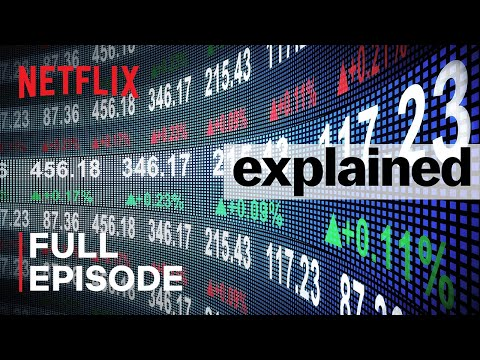# Expectation And Trading On The Stock Exchange

## Video: Expectation And Trading On The Stock ExchangeVideo: Explained | The Stock Market | FULL EPISODE | Netflix 2023, January

Competent trading on stock exchanges can be very profitable. At the same time, it is very important to trade according to the system, observing certain rules. The quality of a trading system is determined by many parameters, one of them is the mathematical expectation.

You cannot trade in the foreign exchange and securities markets without a carefully built trading system. Trading strategies can be different, while their profitability will differ. To assess the profitability of the system, the concept of mathematical expectation was introduced.

## The mathematical expectation of the trading system

The mathematical expectation of the trading system can be greater or less than 0. If the mathematical expectation is above 0, then the system makes a profit. This does not mean that every trade you make will be profitable. But on a large number of transactions, the system will give real profit. The higher the mathematical expectation of a trading system, the greater the profitability.

The same applies to a system with an expectation less than 0, but the result will be the opposite. Some trades made using such a system may be successful, but in the long run the system will be unprofitable. You cannot trade using such a system.

## Calculating the expected value

Expected value is calculated using the following formula: M = P + × V + - P- × V-.

Here "P +" is the probability of profit per 1 trade, calculated as the ratio of the number of profitable trades to their total number. "V +" - the value of the average profit per 1 trade. It is calculated as the ratio of total profit to the total number of transactions. "P-" - the probability of a loss per 1 trade, calculated as the ratio of the number of unprofitable deals to their total number. Finally, “V-” is the average loss per 1 trade, equal to the ratio of the total loss to the total number of deals.

For the calculations to be correct, at least a hundred transactions are required. Expected value can be calculated both on the basis of real trading and by running the system on the tester - it is, for example, in the popular trading terminal Meta Trader 4. But first the rules of the system must be formalized, for this a so-called trading advisor is written - a small program capable of independently open and close transactions according to a given algorithm. The Expert Advisor is run on the trading history, and a lot of data is displayed in the report on its operation, including the expected value.

## How to increase the mathematical expectation

The only way to increase it is to optimize the trading rules. A lot of points are taken into account, among the main ones are the Stop Loss and Take Profit levels, a more accurate determination of the points of entry into the market and exit from it. Unfortunately, it is simply impossible to take into account many elements of a trading system in an EA, therefore, in practice, the effectiveness of a trading system usually has to be determined based on the results of real trading or trading on a demo account.

To determine the expectation in the Meta Trader 4 trading terminal, it is enough to order a report for a certain trading period. To do this, open the "Trade history" tab, right-click on it and select "Save as detailed report".